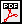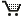# Bi-level Strategies in Semi-infinite Programming

Oliver Stein

Abstract. Semi-infinite optimization in its general form has recently attracted a lot of attention, not only because of its surprising structural aspects, but also due to the large number of applications which can be formulated as general semi-infinite programs.

This is the first book which exploits the bi-level structure of semi-infinite programming systematically. It highlights topological and structural aspects of general semi-infinite programming, formulates powerful optimality conditions which take this structure into account, and gives a conceptually new bi-level solution method.

The results are motivated and illustrated by a number of problems from engineering and economics that give rise to semi-infinite models, including (reverse) Chebyshev approximation, minimax problems, robust optimization, design centering, defect minimization problems for operator equations, and disjunctive programming.

The book is addressed to graduate students and researchers which work in the fields of optimization and operations research. Notions which are necessary for understanding the material but which are not part of the standard university curriculum are briefly explained.Book review by D. Klatte, Mathematical Methods of Operations Research, Vol. 60 (2004), 163.Order your copy at the publisher's web page.